# 5. Product, Coproduct, Exponential¶

## 5.1. Product¶

There is also a categorical version of the Cartesian product of sets. This is another example of a universal property.

Given two objects $$A$$ and $$B$$ a product of $$A$$ and $$B$$ is an object $$P$$ with morphisms $$p_1\colon P\to A$$ and $$p_2\colon P\to B$$ such that for every object $$X$$ and all morphisms $$x_1\colon X\to A$$ and $$x_2\colon X\to B$$ there exists a unique morphism $$h\colon X\to P$$ such that $$p_1\circ h=x_1$$ and $$p_2\circ h=x_2$$.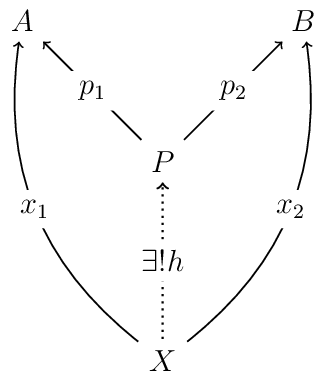If the identities $$p_1\circ h=x_1$$ and $$p_2\circ h=x_2$$ hold, we say, “the diagram in the figure commutes” or “is a commuting diagram” (verb form), or “is commutative” (when we prefer an adjective).

We will make the notions diagram and commutative diagram precise later, once we have acquired the tools necessary to do so. For now, suffice it to adopt the following informal understanding of commuting diagrams.

A commutative diagram is a diagram with the following property: for all objects $$C$$ and $$D$$, all paths from $$C$$ to $$D$$ yield the same morphism.

More precisely, a diagram commutes iff $$\forall x \in C$$, $$\varphi(x) = \psi(x)$$ whenever $$\varphi = f_1 \circ \dots \circ f_m \colon C \to D$$ and $$\psi = g_1 \circ \dots \circ g_n \colon C \to D$$ are compositions of morphisms in the diagram with the same source and target.

Exercise 5.1.1: Verify that the Cartesian product is a product in the category Set.
Exercise 5.1.2: Find a category with two objects which have no product.

Claim: The product (if it exists) is unique up to isomorphism. Suppose that $$(P,p_1,p_2)$$ and $$(Q,q_1,q_2)$$ are two products of $$A$$ and $$B$$. We exhibit an isomorphism between $$P$$ and $$Q$$.

Since $$P$$ is a product there exists a unique morphism $$h\colon Q\to P$$ such that $$p_1\circ h=q_1$$ and $$p_2\circ h=q_2$$. Similarly, since $$Q$$ is a product there exists a unique morphism $$k\colon P\to Q$$ such that $$q_1\circ k=p_1$$ and $$q_2\circ k=p_2$$. See the figure below.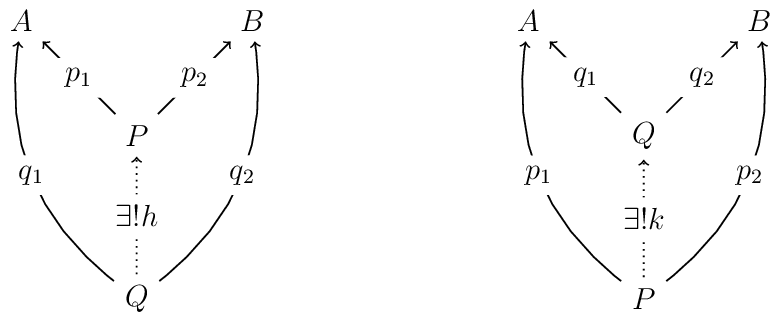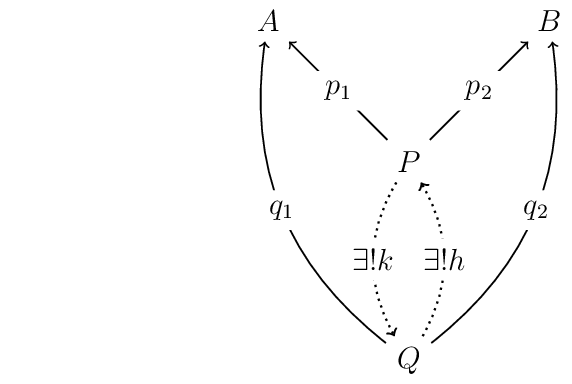We now show that $$h$$ and $$k$$ are inverses of one another. That is, we show $$h\circ k=\operatorname{id}_P$$ and $$k\circ h=\operatorname{id}_Q$$.

Define $$\varphi = h\circ k$$. Then, since

$p_i\circ(h\circ k)=(p_i\circ h)\circ k=q_i\circ k=p_i, \text{ for each } i \in \{1,2\}.$

the following diagram is commutative: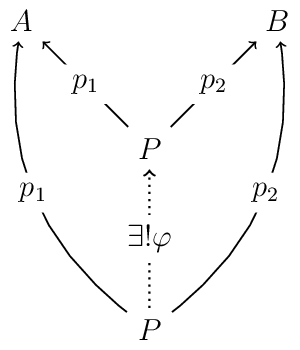On the other hand, taking $$\varphi = \operatorname{id}_P$$ obviously results in a commuting diagram as well.

Therefore, by uniqueness of $$\varphi$$, we obtain $$h\circ k=\operatorname{id}_P$$.

Of course, the same argument with the roles of $$P$$ and $$Q$$ exchanged yields $$k\circ h=\operatorname{id}_Q$$ also holds.

We write $$A\times B$$ to denote the product of $$A$$ and $$B$$. We usually use $$\pi_1 \colon A\times B \to A$$ and $$\pi_2 \colon A \times B \to B$$ to denote the projections and $$\left\langle x_1, x_2 \right\rangle$$ for the unique map $$h\colon X\to A\times B$$.

In the category $$\mathbf{Rel}$$ of relational structures, the product is the disjoint union. We will return to this example below, once we define the coproduct.

Exercise 5.1.3: Verify that the diagram commutes and that $$⟨r, s⟩$$ is unique.
Exercise 5.1.4: What are the products in Grph, Mon, and Pos?

## 5.2. Coproduct¶

The dual notion to the product is the coproduct.

Given two objects $$A$$ and $$B$$ in a category $$\mathcal C$$ the coproduct (or sum) of $$A$$ and $$B$$ is denoted $$A+B$$ and defined to be the object in $$\mathcal C$$ with morphisms from $$A$$ and $$B$$ into itself that satisfy the following universal property:

If these morphisms are denote by $$\mathrm{inl} : A → A + B$$ and $$\mathrm{inr} : B → A + B$$, then for every object $$X$$ and all morphisms $$u : A → Y$$ and $$v : B → Y$$ there exists a unique morphism $$[u,v] : A + B → Y$$ such that $$[u,v] ∘ \mathrm{inl} = u$$ and $$[u,v] ∘ \mathrm{inr} = v$$, as illustrated in the diagram below.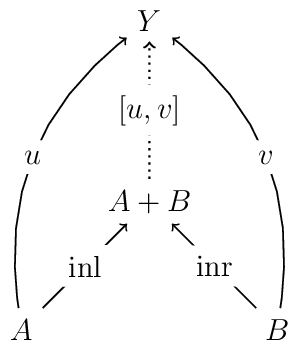In $$\mathbf{Set}$$ the sum is the disjoint union. That is, $$A+B=A\uplus B$$.

In $$\mathbf{Rel}$$ sums are also disjoint unions. This follows from $$\mathbf{Rel}$$ being self-dual.

The morphisms $$\mathrm{inl}$$ and $$\mathrm{inr}$$ attach to each element of $$A + B$$ with a tag that tells us from which object, $$A$$ or $$B$$, did the element come.

For instance, if $$x : A + B$$, then either $$x$$ denotes an element from $$A$$, say, $$a :A$$, in which case $$x = \mathrm{inl} a$$, or else $$x$$ denotes an element $$b : B$$, in which case $$x = \mathrm{inr} b$$.

This is important especially in case we wish to form a disjoint union of, say, two sets that have some elements in common. For example, Let $$A = \{0,1,2\}$$ and $$B = \{2, 3\}$$. Even though these sets are not disjoint, since $$A ∩ B = \{2\}$$, we can still form the disjoint union.

$A + B = \{\mathrm{inl} 0, \mathrm{inl} 1, \mathrm{inl} 2, \mathrm{inr} 2, \mathrm{inr} 3\}.$

More generally given two objects $$A$$ and $$B$$ in a category $$\mathcal C$$, the coproduct of $$A$$ and $$B$$ is the object

$A + B = \{\mathrm{inl} a : a ∈ A\} ∪ \{\mathrm{inr} b : b ∈ B\}.$

For some categories the coproduct is the product. As we mentioned above, this is the case for the category $$\mathbf{Rel}$$ of relational structures. Indeed, here is a diagram illustrating the (co)product in $$\mathbf{Rel}$$.The figure above displays the commutative diagram. The unique morphism that makes this diagram commute is $${\left\langle r,s \right\rangle}=\{(x,\mathrm{inl} a) : x\mathrel{r} a\}\cup \{(x,\mathrm{inr} b) : x\mathrel{s} b\}$$.

Exercise 5.2.1: Prove that the coproduct is unique up to isomorphism.
Exercise 5.2.2: What are the sums in Grph, Mon, and Pos?

## 5.3. Exponential¶

### 5.3.1. Internal reflection¶

Given two objects $$A$$ and $$B$$ in a category, consider the object $$B^A$$.

This “represents internally” the class of all morphisms from $$A$$ to $$B$$.

(Some authors denote $$B^A$$ by $$A \Rightarrow B$$, or even $$A \to B$$.)

Let $$\mathcal C$$ be a category with (binary) products and let $$f_1 \colon A_1 \to B_1$$ and $$f_2 \colon A_2 \to B_2$$ be morphisms.

The product morphism of $$f_1$$ and $$f_2$$ is denoted by $$f_1 \times f_2 : A_1 \times A_2 \to B_1 \times B_2$$ and defined by

$f_1 \times f_2 = \langle f_1 \circ \pi_1, f_2 \circ \pi_2\rangle.$

The product of $$f_1$$ and $$f_2$$ is diagramed as follows: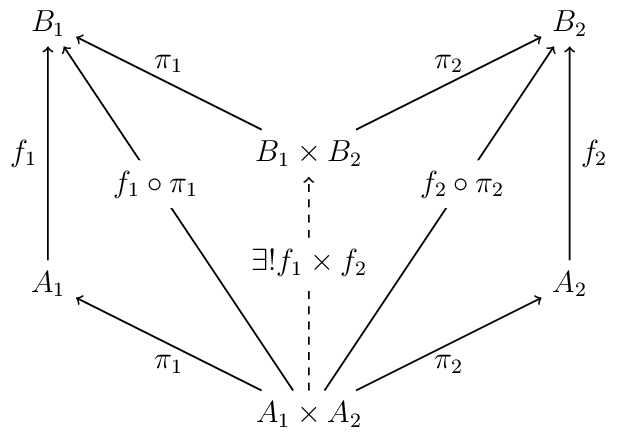Again, let $$\mathcal C$$ be a category where all binary products exist.

Given two objects $$A$$ and $$B$$ from $$\mathcal C$$, an object $$C$$ is called an exponential from $$A$$ to $$B$$ iff there exists a morphism $$eval^A_B\colon C\times A\to B$$ satisfying the following condition:

• for every object $$X$$ and morphism $$f\colon X\times A\to B$$ there is a unique morphism $$\lambda f\colon X\to C$$ such that $$eval^A_B\circ(\lambda f\times\mathrm{id}_A)=f$$ (so the diagram at right in the figure below commutes).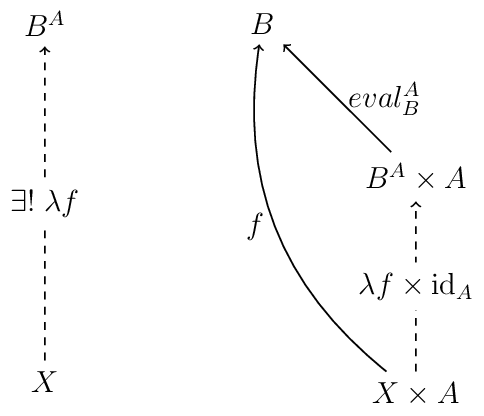Exercise 5.3.1: Show that the exponential is unique up to isomorphism.

Example 5.1

In $$\mathbf{Set}$$, the exponential is just the set of functions from $$A$$ to $$B$$:

$B^A = \mathrm{Hom}_{\mathbf{Set}}(A, B)$

where $$eval : B^A \times A \to B$$ is defined for each $$(g, a) \in B^A \times A$$ by

$eval(g, a) = g(a)$

and $$\lambda f : X \to B^A$$ is defined for each $$X$$ and $$f : X \times A \to B$$ by

$\lambda f x : a \mapsto f(x,a)$

Exercise 5.3.2: Verify that $$B^A$$ satisfies the definition of an exponential with the morphisms $$eval$$ and $$λ f$$. In addition to checking the requisite equalities be sure to show that $$λ f$$ is unique.
Exercise 5.3.3: Does the category Mon have exponential objects?

Example 5.2

The category $$\mathbf{Pos}$$ of partially ordered sets (or “posets”) has exponential objects. Given posets $$P$$ and $$Q$$ we define

$Q^P = \{f\colon P\to Q\mid f\text{ is monotone}\}.$

Exercise 5.3.4: Define $$eval$$ and $$λ$$ for Pos and prove that $$Q^P$$ is an exponential object with respect to these morphisms.

In categories whose objects are sets with extra structure the exponentials are often defined by taking the set of morphisms between two objects and giving it a structure inherited from the codomain. (What happens if one tries to do this in $$\mathbf{Mon}$$?)

Example 5.3

In the category $$\mathbf{Grph}$$ the exponential is not just the set of morphisms from one object to another.

Given two graphs $$\mathcal G$$ and $$\mathcal H$$ the exponential graph $$\mathcal H^{\mathcal G}$$ has

• Vertices. $$V = V_{\mathcal G} \to V_{\mathcal H}$$ (all maps from the vertices of $$\mathcal G$$ to the vertices of $$\mathcal H$$);
• Edges. An edge $$\alpha$$ from a vertex $$f$$ to a vertex $$g$$ in $$\mathcal H^{\mathcal G}$$ is a function $$\alpha\colon E_{\mathcal G}\to E_{\mathcal H}$$ such that for every edge $$(a,b)\in E_{\mathcal G}$$ we have $$(f(a),g(b))\in E_{\mathcal H}$$.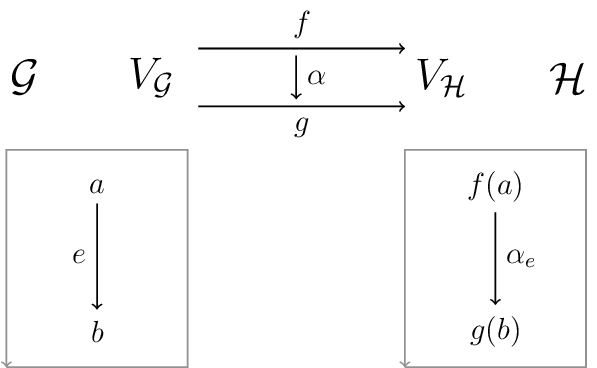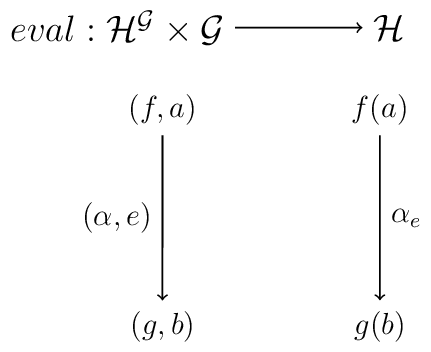If $$f \colon \mathcal K \times \mathcal G \to \mathcal H$$, define $$\lambda f \colon \mathcal K \to \mathcal H^{\mathcal G}$$ as in the following diagram: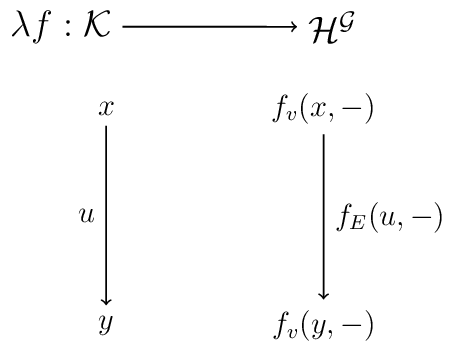Exercise 5.3.5: Verify that the above definition of "exponential graph" is indeed that of the exponential object in Grph.
Exercise 5.3.6: Can you define exponentials in Par and Rel?

## 5.4. Cartesian closed category¶

We now have the language to discuss a special kind of category.

A Cartesian closed category (CCC) is a category with a terminal object, binary products, and exponentials.

Note that we have already done the work to show that $$\mathbf{Set}$$, $$\mathbf{Pos}$$, and $$\mathbf{Grph}$$ are Cartesian closed.

For all CCCs we have the following result.

In a Cartesian closed category $$\mathcal C$$, for each triple of objects, $$A$$, $$B$$, $$C$$, we have the following isomorphism of hom-sets:

$\mathrm{Hom}_{\mathcal C}(C\times A, B)\cong \mathrm{Hom}_{\mathcal C}(C, B^A),$

(Here $$\cong$$ simply denotes bijection of sets; i.e., $$\mathbf{Set}$$-isomorphism.)

From left to right, the isomorphism is given by the $$\lambda$$ operation of exponentials.

From right to left, the isomorphism is given by application morphism $$ap \colon\mathrm{Hom}_{\mathcal C}(C,B^A)\to\mathrm{Hom}_{\mathcal C}(C\times A,B)$$. This is the map that takes each $$g \in \mathrm{Hom}_{\mathcal C}(C, B^A)$$, to the morphism $$ap(g)\colon C \times A \to B$$ defined by $$ap(g) = eval \circ(g\times\mathrm{id}_A)$$.

Exercise 5.4.1: Show that $$λ$$ and $$ap$$ do indeed give set isomorphisms, establishing the proposition. (Draw the appropriate diagrams!)
Exercise 5.4.2: Let $$\mathbf{1}$$ be the terminal object in a CCC. Prove that $$\mathbf{1} × A ≅ A$$, for every object $$A$$.
Exercise 5.4.3: Let $$A_1 ≅ A_2$$ in a CCC. Show that $$\mathrm{Hom}_{\mathcal C}(A_1,B) ≅ \mathrm{Hom}_{\mathcal C}(A_2,B)$$ and similarly for the codomain (i.e., $$\mathrm{Hom}_{\mathcal C}(B, A_1) ≅ \mathrm{Hom}_{\mathcal C}(B, A_2)$$). (Note that $$A_1 ≅ A_2$$ is isomorphism of objects in the category $$\mathcal C$$, while the second isomorphism is bijection of sets.)

We then have

$\mathrm{Hom}(A,B)\cong\mathrm{Hom}(\mathbf{1}\times A,B)\cong\mathrm{Hom}(\mathbf{1},B^A),$

so the morphisms from $$A$$ to $$B$$ are in one-to-one correspondence with the global points of $$B^A$$.

In $$\mathbf{Set}$$ this says that the elements of $$B^A$$ are the functions from $$A$$ to $$B$$, as expected.

In $$\mathbf{Grph}$$ this says that the morphisms from the graph $$A$$ to the graph $$B$$ are the loops of $$\mathcal B^{\mathcal A}$$!

Exercise 5.4.4: What does this say about Mon, Par, and Rel? (Remember, in those categories $$\mathbf{1} = \mathbf{0}$$!)

## 5.5. Solutions¶

Exercise 5.1.1

Solution (to do).

Exercise 5.1.2

Solution (to do).

Exercise 5.1.3

Solution (to do).

Exercise 5.1.4

Solution (to do).

Exercise 5.2.1

Solution (to do).

Solution to Exercise 5.2.2.

Fix $$f$$ a morphism of Set. To prove $$Kf$$ is a morphism of Mon, we must show that, as a function from $$KA = A^∗$$ to $$KB = B^∗$$, $$Kf$$ preserves the monoid structure. That is, if $$w, w' \in A^\ast$$.

Indeed, if $$w = a_0 \cdots a_n$$ and $$w' = a'_0\cdots a'_n$$, then

$\begin{split}Kf(w :: w') &= map(f)(w :: w') \\ &= map(f)(a_0\cdots a_n a'_0\cdots a'_n)\\ &= f(a_0)\cdots f(a_n)f(a'_0)\cdots f(a'_n)\\ &= f(a_0)\cdots f(a_n)::f(a'_0)\cdots f(a'_n)\\ &= map(f)(a_0\cdots a_n) :: map(f)(a'_0\cdots a'_n)\\ &= Kf(w) :: Kf(w').\end{split}$

Exercise 5.3.1

Solution (to do).

Exercise 5.3.2

Solution (to do).

Exercise 5.3.3

Solution (to do).

Exercise 5.3.4

Solution (to do).

Exercise 5.3.5

Solution (to do).

Exercise 5.3.6

Solution (to do).

Exercise 5.4.1

Solution (to do).

Exercise 5.4.2

Solution (to do).

Exercise 5.4.3

Solution (to do).

Exercise 5.4.4

Solution (to do).